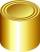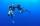# Megapascals

What is the area of crosssection of the piston, if the force of 300 kN produces a pressure of 5 MPa?

Result

S =  600 cm2

#### Solution:Leave us a comment of example and its solution (i.e. if it is still somewhat unclear...):

Showing 0 comments:Be the first to comment!## Next similar examples:

1. GardenThe garden around the new majer is divided as follows: 35% vegetable, 30% fruit orchards, 10% flowers and the remaining 120 m2 are lawns. What is the total area of the garden?
2. Reservoir6 m long reservoir has a diameter of 2.2 m. What is its surface area in square meters?
3. DiameterWhat is the inside diameter of the cylinder container and if half a liter of water reaches a height 15 cm?
4. Diver and pressureDetermine what pressures is exposed diver without a suit at a depth of 15 meters, with a small space suit at a depth of 25 meters and a massive suit at a depth of 70 m in water.
5. ClassIn a class are 32 pupils. Of these are 8 boys. What percentage of girls are in the class?
6. Pipe cross sectionThe pipe has an outside diameter 1100 mm and the pipe wall is 100 mm thick. Calculate the cross section of this pipe.
7. RhombusPQRS is a rhombus. Given that PQ=3 cm & height of rhombus is given 2 cm. Calculate its area.
8. PipeHow long is the pipe with an outside diameter of 1.44 m if his coloring consumed 44 kg of color. 1 kg of color coverage is 9 m2.
9. Trapezium 2Trapezium has an area of 24 square cms. How many different trapeziums can be formed ?
10. GreenhouseGarden plastic greenhouse is shaped half cylinder with a diameter of 6 m and base length 20 m. At least how many m2 of plastic is need to its cover?
11. Area of ditchHow great content area will have a section of trapezoidal ditch with a width of 1.6 meters above and below 0.57 meters? The depth of the ditch is 2.08 meters.
12. TrapeziumThe area of trapezium is 35cm2 find its altitude if the bases are 6cm and 8cm.
13. MO 2016 Numerical axisCat's school use a special numerical axis. The distance between the numbers 1 and 2 is 1 cm, the distance between the numbers 2 and 3 is 3 cm, between the numbers 3 and 4 is 5 cm and so on, the distance between the next pair of natural numbers is always in
14. InequationSolve the inequation: 5k - (7k - 1)≤ 2/5 . (5-k)-2
15. Down syndromeDown syndrome is one of the serious diseases caused by a gene mutation. Down syndrome occurs in approximately every 550-born child. Express the incidence of Down's syndrome in newborns at per mille.
16. The percentages in practiceIf every tenth apple on the tree is rotten it can be expressed by percentages: 10% of the apples on the tree is rotten. Tell percent using the following information: a. in June rained 6 days b, increase worker pay 500 euros to 50 euros c, grabbed 21 fro
17. Base, percents, valueBase is 344084 which is 100 %. How many percent is 384177?